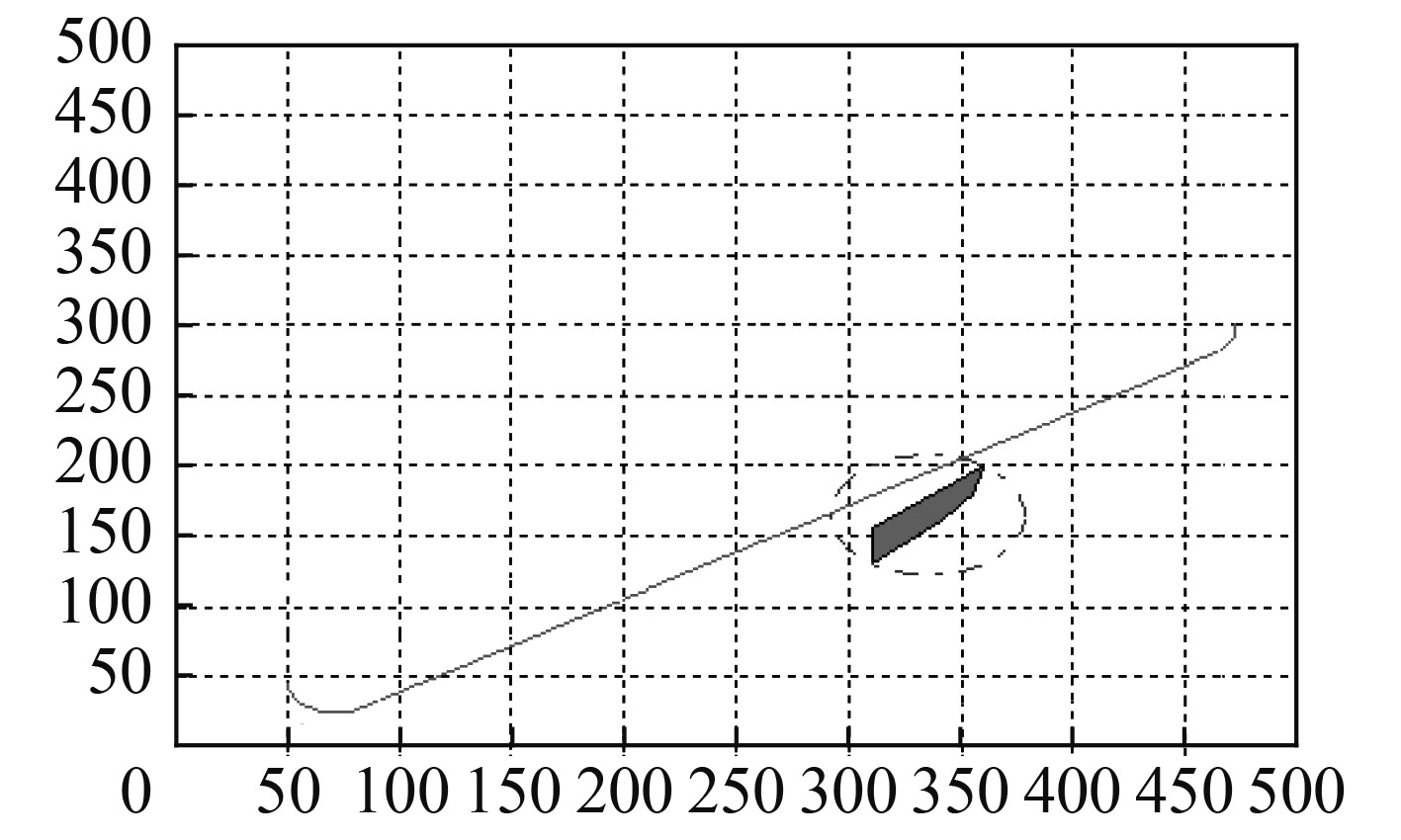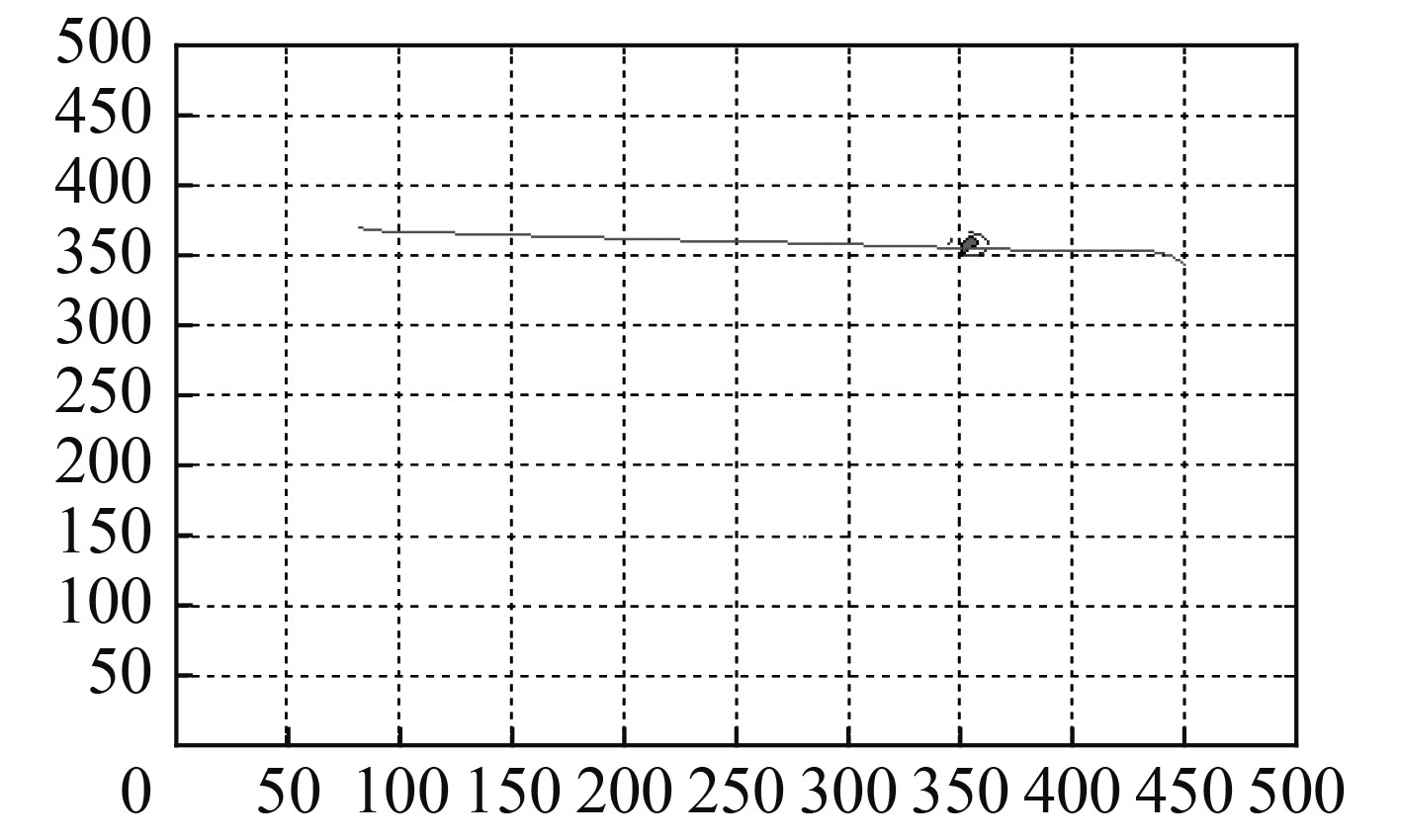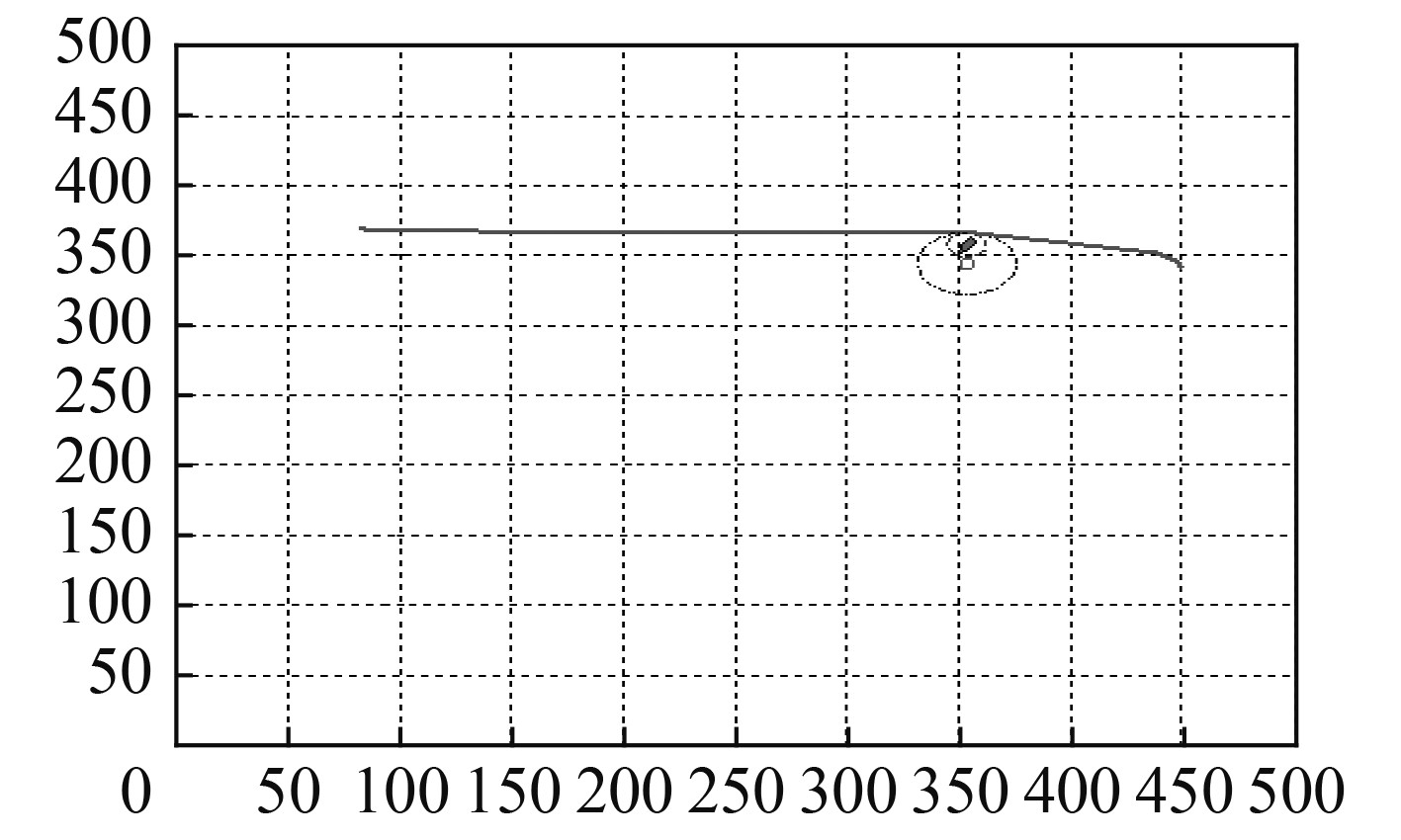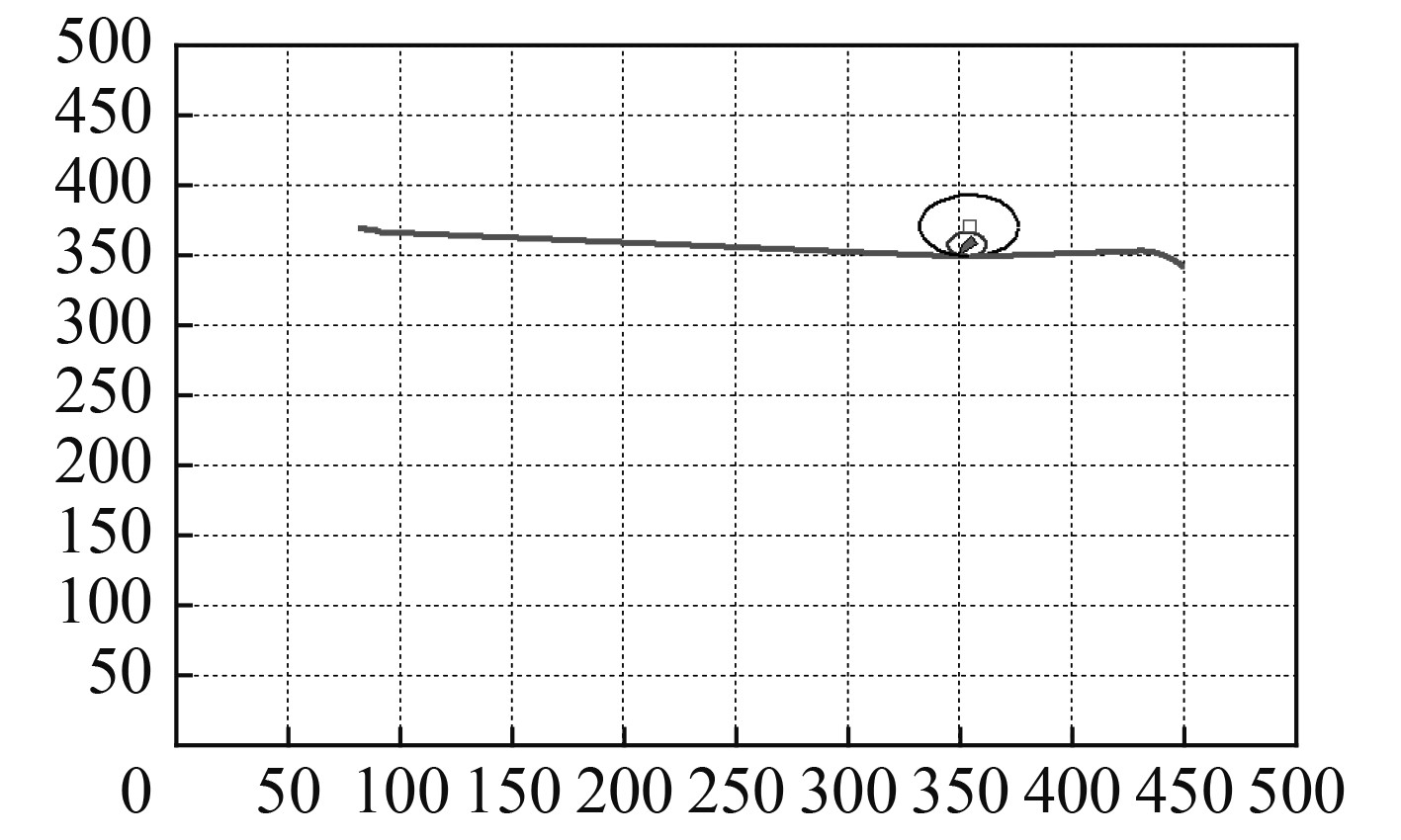﻿ 一种改进的基于Clothoid曲线的水下无人航行器路径规划方法
 舰船科学技术2020, Vol. 42Issue (6): 85-88    DOI: 10.3404/j.issn.1672-7649.2020.06.017PDF

1. 海军研究院博士后科研工作站，北京 100161;
2. 北京邮电大学 网络教育学院，北京 100088;
3. 海军研究院，北京 100161

An improved path planning method for UUV based on clothoid curves
LAN Xiao-juan1,2, CHEN Qiang3
1. Postdoctoral Research Workstation, Naval Research Academy, Beijing 100161, China;
2. School of Network Education, Beijing University of Posts and Telecommunications, Beijing 100088, China;
3. Naval Research Academy, Beijing 100161, China
Abstract: In order to plan a obstacle-free short distance path from the starting point to the target point on the chart, which is easy for Unmanned Underwater Vehicle(UUV) to realize close tracking, and solve problems in the obstacle avoidance method for UUV’s path planning based on Clothoid curves, two improvement measures are proposed: (1)When the path intersects the obstacle circle, it is necessary to judge whether the path intersects the boundary of the obstacle. If the system detects that the path does not intersect the boundary, there is no need to draw a new path to avoid the obstacle. (2) When the curvature of the obstacle circle doesn’t meet kinematic constraints, a new obstacle circle which is internal tangent with the original obstacle circle is generated according to the kinematic constraint curvature. Theoretical analysis and simulation results show that, the new path generated according to the improvement measures avoids drawing some unnecessary obstacle avoidance paths, meets kinematic constraints, and is easy to achieve.
Key words: unmanned underwater vehicle     clothoid curves     path planning     obstacle avoidance
0 引　言

2个位姿点间的最短路径是Dubins路径，Dubins 路径由 Dubins 在 1957 年完成数学证明。Dubins路径被定义为：在最大曲率限制下，平面内2个有方向的点间的最短可行路径是CLC路径或CCC路径，或是它们的子集，其中C表示圆弧段，L表示与C相切的直线段。Dubins路径的几何特性简单，但是当路径由圆弧段过渡到直线段或由直线段过渡到圆弧段时，路径上曲率变化不连续，会使水下无人航行器的路径跟踪产生较大误差。曲率随路径长度线性变化的Clothoid 曲线可使路径在直线段与圆弧段间有平滑的过渡，所以基于Clothoid 曲线的复合路径更易于水下无人航行器实现紧密跟踪

1 传统Clothoid复合路径避障算法的设计

Clothoid 曲线是一种螺旋曲线，通过计算Fresnel积分得到，路径的曲率随曲线弧长线性变化。路径的方向需要沿路径长度对曲率积分：

 $\phi (q) = \int_0^q {\kappa \frac{k}{h}} {\rm{d}} k = \frac{\kappa }{{2h}}{q^2}\text{，}$ (1)

 \left\{ {\begin{aligned} & {x(h) = \int_0^h {\cos (\frac{\kappa }{{2h}}{q^2}){\rm{d}}q} }\text{，}\\ & {y(h) = \int_0^h {sin(\frac{\kappa }{{2h}}{q^2}){\rm{d}}q} }\text{。} \end{aligned}} \right. (2)

1）路径的直线段与一个障碍物圆相交

 $\frac{{{d_n}}}{{{r_o}}} < 1,{\rm{ }}{d_n}{\kappa _o} < 1\text{。}$ (3)

2）路径的弧线段与障碍物圆相交

 \left\{ \begin{aligned} & \frac{1}{{{\kappa _{\rm{S}}}_1}}{\rm{ + }}\frac{1}{{{\kappa _{\rm{o}}}}} > {d_{\rm{c}}} > \frac{1}{{{\kappa _{\rm{S}}}_1}} - \frac{1}{{{\kappa _{\rm{o}}}}}\text{，}\\ & \frac{1}{{{\kappa _{\rm{S}}}_2}}{\rm{ + }}\frac{1}{{{\kappa _{\rm{o}}}}} > {d_{\rm{c}}} > \frac{1}{{{\kappa _{\rm{S}}}_2}} - \frac{1}{{{\kappa _{\rm{o}}}}}\text{。} \end{aligned} \right. (4)

1）当Clothoid路径与障碍物圆相交时，不一定与障碍物本身相交，如果按相交情况处理，就需要重新画避障路径，不仅增加了计算量，而且还增加了Clothoid路径的长度；

2）当Clothoid路径直线段与障碍物相交时，障碍物圆的曲率不一定满足水下无人航行器的运动约束，此时按原障碍物圆设计的Clothoid避障路径不满足运动约束，在实际情况中无法实现。

2 对Clothoid路径避障算法的改进

1）当Clothoid路径与障碍物圆相交时，检测与障碍物边界是否相交。

 \left\{ \begin{aligned} & {d_{ip}} = {d_o}\cos {\theta _o} + \sqrt {{d_o}^2({{\cos }^2}{\theta _o} - 1) + \frac{1}{{{\kappa _o}^2}}}\text{，} \\ & {d_{im}} = {d_o}\cos {\theta _o} - \sqrt {{d_o}^2({{\cos }^2}{\theta _o} - 1) + \frac{1}{{{\kappa _o}^2}}} \text{。} \end{aligned} \right. (5)

 \left\{ \begin{aligned} & {\left( {{x_1}({h_1}) - {x_z}} \right)^2}{\rm{ + }}{\left( {{y_1}({h_1}) - {y_z}} \right)^2} = \frac{1}{{{\kappa _o}^2}}\text{，}\\ & {\left( {{x_2}({h_2}) - {x_z}} \right)^2}{\rm{ + }}{\left( {{y_2}({h_2}) - {y_z}} \right)^2} = \frac{1}{{{\kappa _o}^2}}\text{。} \end{aligned} \right. (6)

2）当Clothoid路径直线段与障碍物相交时，如果障碍物圆的曲率不满足运动约束，按照运动约束重新画障碍物圆。

①将原障碍物圆全部包括在内；

②与原障碍物圆内切；

③满足运动约束曲率。

 \left\{ \begin{aligned} & {({x_q} - {x_0})^2} + {({y_q} - {y_0})^2} = \frac{1}{{{\kappa _n}^2}}\text{，}\\ & {(x - {x_0})^2} + {(y - {y_0})^2} = \frac{1}{{{\kappa _n}^2}}\text{，}\\ & \frac{{{x_0} - {x_z}}}{{{y_0} - {y_z}}} = - \frac{{{y_{{P_X}}} - {y_q}}}{{{x_{{P_X}}} - {x_q}}}\text{。} \end{aligned} \right. (7)

1）生成的新障碍物圆将原障碍物圆全部包括，确保了生成的新路径不会与原障碍物圆内的障碍物相交；

2）改进的新路径不改变起始弧线段的曲率，直线段起始点和起始点到障碍物圆的直线段与使用原障碍物圆时相同，减少了计算量。

3 Clothoid路径规划方法仿真

1）水下无人航行器从起始位姿点[50, 48, 90°]以2 kn的速度航行到终止位姿点[472, 300, 90°]。从图1可以看出，起始位姿点与终止位姿点的连线与1个障碍物圆相交，但是没有与障碍物圆内的障碍物边界相交，因此根据检测结果不需要重新画Clothoid路径（由于横坐标与纵坐标显示比例不一致，障碍物圆显示结果呈椭圆形）。图 1 没有与障碍物相交的Clothoid路径 Fig. 1 A Clothoid path without intersecting with the obstacle

2）水下无人航行器从起始位姿点[82, 370, 50°]以4 kn的速度航行到终止位姿点[450, 341, 80°]。从图2可以看出，起始位姿点与终止位姿点的原始路径与1个障碍物圆相交，障碍圆的半径小于水下无人航行器的转弯半径，因此根据水下无人航行器的运动约束运用改进的Clothoid路径规划方法生成避障路径。生成的2条避障路径分别如图3图4所示。图3中的公切圆为顺时针旋转生成，图4中的公切圆为逆时针旋转生成。图 2 与障碍物相交的Clothoid路径 Fig. 2 A Clothoid path intersecting with the obstacle图 3 顺时针旋转生成新障碍物圆的Clothoid路径 Fig. 3 A Clothoid path with a new clockwise obstacle circle图 4 逆时针旋转生成新障碍物圆的Clothoid路径 Fig. 4 A Clothoid path with a new anticlockwise obstacle circle
4 结　语

1）当Clothoid路径与障碍物圆相交时，计算与障碍物圆的交点，然后检测与障碍物圆相交的2个交点间的直线段或弧线段是否与障碍物的边界线相交，如果没有相交，就不需要画避障路径。

2）当Clothoid路径直线段与障碍物相交时，如果障碍物圆的曲率不满足水下无人航行器的运动约束，按照运动约束生成将原障碍物圆全部包括的内切障碍物圆。

  孙兵, 朱大奇, 杨元元. 基于粒子群优化的自治水下机器人模糊路径规划[J]. 高技术通讯, 2013, 23(12): 1284-1291. SUN Bing, ZHU Daqi, YANG Yuanyuan. Fuzzy path planning for autonomous underwater vehicles based on particle swarm optimization[J]. High Technology Letters, 2013, 23(12): 1284-1291. DOI:10.3772/j.issn.1002-0470.2013.12.011  高剑, 李勇强, 徐德民, 等. 基于行为的自主水下航行器无碰路径跟踪控制[J]. 大连海事大学学报, 2012, 38(4): 30-34. GAO Jian, LI Yong-qiang, XU De-min, et al. Behavior-based collision free path following control of an autonomous underwater vehicle[J]. Journal of Dalian Maritime University, 2012, 38(4): 30-34.  王丹. 基于粒子群优化的多路径规划方法研究[J]. 舰船科学技术, 2011, 33(6): 156-159. WANG Dan. Research on multi-path planning method based on particle swarm optimization[J]. Ship Science and Technology, 2011, 33(6): 156-159. DOI:10.3404/j.issn.1672-7649.2011.06.036  姜岩, 龚建伟, 熊光明, 等. 基于运动微分约束的无人车辆纵横向协同规划算法的研究[J]. 自动化学报, 2013, 39(12): 2012-2020. JIANG Yan, GONG Jian-Wei, XIONG Guang-Ming, et al. Research on differential constraints-based planning algorithm for autonomous-driving vehicle[J]. Acta Automatica Sinica, 2013, 39(12): 2012-2020.  CASTRO A. L. , KOILLER J.. On the dynamic Markov-Dubins problem: From path planning in robotics and biolocomotion to computational anatomy[J]. Regular and Chaotic Dynamics, 2013, 18(1-2): 1-20. DOI:10.1134/S1560354713010012  梁勇, 张友安, 雷军委. 一种基于Dubins路径的在线快速航路规划方法[J]. 系统仿真学报, 2013, 25(S1): 291-296. LIANG Yong, ZHANG You-an, LEI Jun-wei. New method of online fast path planning based dubins path[J]. Journal of System Simulation, 2013, 25(S1): 291-296.  王怿, 祝小平, 周洲. 一种基于 Clothoid曲线的无人机路径规划算法[J]. 西北工业大学学报, 2012, 30(6): 874-878. WANG Yi, ZHU Xiaoping, ZHOU Zhou. A better path planning algorithm based on clothoid curves for unmanned aerial vehicles(UAVs)[J]. Journal of Northwestern Polytechnical University,, 2012, 30(6): 874-878. DOI:10.3969/j.issn.1000-2758.2012.06.014  MADHAVAN S, ANTONIOS T, BRIAN W, et al. Co-operative path planning of multiple UAVs using Dubins paths with clothoid arcs[J]. Control Engineering Practice, 2010, 18(9): 1084-1092. DOI:10.1016/j.conengprac.2009.02.010